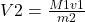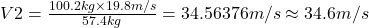## Three kids are riding on a snow sled traveling horizontally without friction at 19.8 m/s. The masses of Kid A, B, and C are 42.8 kg, 31.5 kg

Question

Three kids are riding on a snow sled traveling horizontally without friction at 19.8 m/s. The masses of Kid A, B, and C are 42.8 kg, 31.5 kg, and 25.9 kg, respectively.

What is the new velocity of the sled if Kid A jumps off vertically from the sled? (Kid A velocity = 0)Three kids are riding on a snow sled traveling horizontally without friction at 19.8 m/s. The masses of Kid A, B, and C are 42.8 kg, 31.5 kg, and 25.9 kg, respectively.

What is the new velocity of the sled if Kid A jumps off vertically from the sled? (Kid A velocity = 0)

in progress 0
6 months 2021-08-07T16:16:02+00:00 1 Answers 2 views 0

34.6 m/s

Explanation:

From conservation of momentum, the sum of initial and final momentum are equal. Momentum is a product of mass and velocity. Initial mass will be 42.8+31.5+25.9=100.2 kg

Final mass will be 31.5+25.9=57.4 kg

From formula of momentum

M1v1=m2v2

Making v2 the subject of the formula thenSubstitute 100.2 kg for M1, 19.8 m/s fkr v1 and 57.4 kg for m2 then